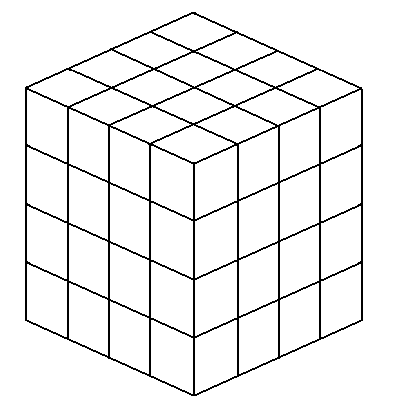## A CUBE OF CUBESIf you pack together a lot of cubes, all the same size, to make another cube you get something like the diagram shown. You can see most of the cubes in the pack and if you are allowed to turn it round you can see even more. But there are some you can't see. The diagram shows a cube of cubes of order 4. The number of cubes in the pack is therefore 4 x 4 x 4 which comes to 64. But you can only see 56, the other eight are hidden by the edge cubes. Those inner eight that you can't see form another cube of cubes, this time of order 2 (2 x 2 x 2 = 8).

A cube of cubes of order 3 would make 26 cubes you could see on the outside and one cube inside that you can't see, and an order 2 cube of cubes, of course, leaves none that you can't see.

The problem this month is to find the order of a cube of cubes that is the smallest order where the number of cubes you cannot see is larger than the number of cubes you can see on the outside.